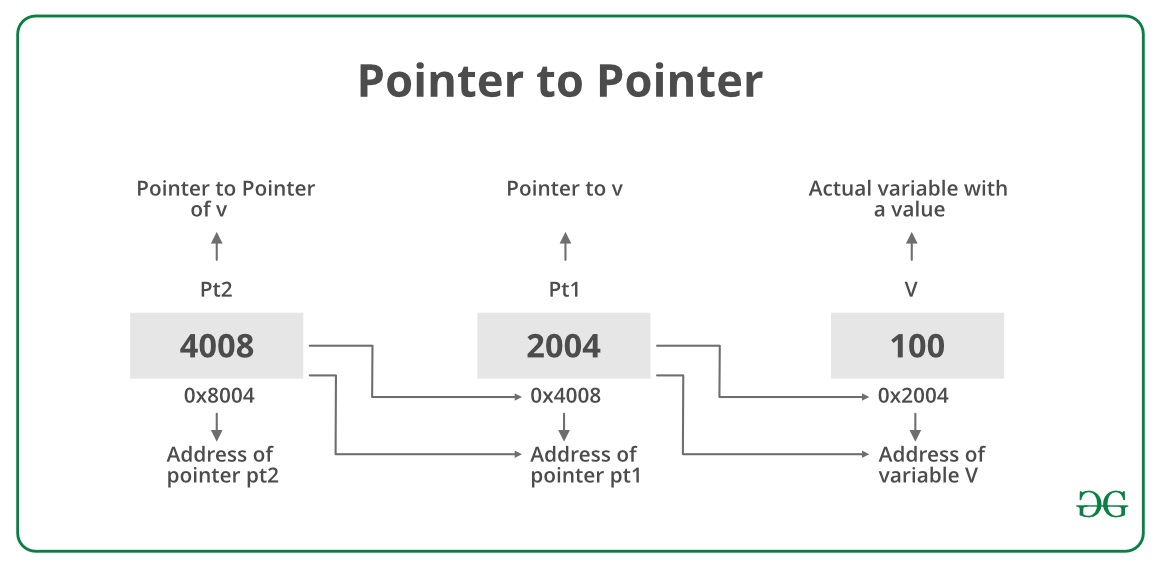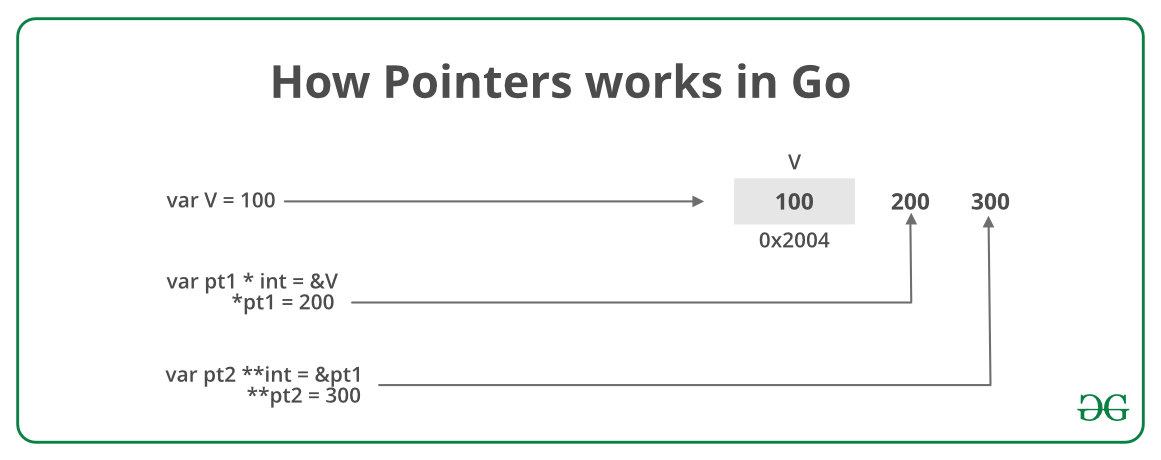Open In App
Related Articles
• Write an Interview Experience
• Golang

# Go Pointer to Pointer (Double Pointer)

Prerequisite: Pointers in Go

Pointers in Go programming language or Golang is a variable which is used to store the memory address of another variable. A pointer is a special variable so it can point to a variable of any type even to a pointer. Basically, this looks like a chain of pointers. When we define a pointer to pointer then the first pointer is used to store the address of the second pointer. This concept is sometimes termed as Double Pointers.

How to Declare a pointer to pointer in Golang?
Declaring Pointer to Pointer is similar to declaring pointer in Go. The difference is we have to place an additional ‘*’ before the name of pointer name. This is generally done when we are declaring the pointer variable using the var keyword along with the type. Below example and image will explain the concept in much better way.

Example 1: In the below program the pointer pt2 stores the address of pt1 pointer. Dereferencing pt2 i.e. *pt2 will give the address of variable v or you can also say the value of pointer pt1. If you will try **pt2 then this will give the value of the variable v i.e. 100.`// Go program to illustrate the``// concept of the Pointer to Pointer``package main``  ` `import ``"fmt"``  ` `// Main Function``func main() {``  ` `        ``// taking a variable``        ``// of integer type``    ``var V ``int` `= 100``      ` `    ``// taking a pointer ``    ``// of integer type ``    ``var pt1 *``int` `= &V``      ` `    ``// taking pointer to ``    ``// pointer to pt1``    ``// storing the address ``    ``// of pt1 into pt2``    ``var pt2 **``int` `= &pt1``  ` `    ``fmt.Println(``"The Value of Variable V is = "``, V)``    ``fmt.Println(``"Address of variable V is = "``, &V)``  ` `    ``fmt.Println(``"The Value of pt1 is = "``, pt1)``    ``fmt.Println(``"Address of pt1 is = "``, &pt1)``  ` `    ``fmt.Println(``"The value of pt2 is = "``, pt2)``  ` `    ``// Dereferencing the ``    ``// pointer to pointer``    ``fmt.Println(``"Value at the address of pt2 is or *pt2 = "``, *pt2)``      ` `    ``// double pointer will give the value of variable V``    ``fmt.Println(``"*(Value at the address of pt2 is) or **pt2 = "``, **pt2)``}`

Output:

```The Value of Variable V is =  100
Address of variable V is =  0x414020
The Value of pt1 is =  0x414020
Address of pt1 is =  0x40c128
The value of pt2 is =  0x40c128
Value at the address of pt2 is or *pt2 =  0x414020
*(Value at the address of pt2 is) or **pt2 =  100
```

Example 2: Let’s make some changes in the above program. Assigning some new value to the pointers by changing the value of pointers using dereferencing as shown below:`// Go program to illustrate the``// concept of the Pointer to Pointer``package main`` ` `import ``"fmt"`` ` `// Main Function``func main() {`` ` `    ``// taking a variable``    ``// of integer type``    ``var v ``int` `= 100`` ` `    ``// taking a pointer``    ``// of integer type``    ``var pt1 *``int` `= &v`` ` `    ``// taking pointer to``    ``// pointer to pt1``    ``// storing the address``    ``// of pt1 into pt2``    ``var pt2 **``int` `= &pt1`` ` `    ``fmt.Println(``"The Value of Variable v is = "``, v)`` ` `    ``// changing the value of v by assigning``    ``// the new value to the pointer pt1``    ``*pt1 = 200`` ` `    ``fmt.Println(``"Value stored in v after changing pt1 = "``, v)`` ` `    ``// changing the value of v by assigning``    ``// the new value to the pointer pt2``    ``**pt2 = 300`` ` `    ``fmt.Println(``"Value stored in v after changing pt2 = "``, v)``}`

Output:

```The Value of Variable v is =  100
Value stored in v after changing pt1 =  200
Value stored in v after changing pt2 =  300
```